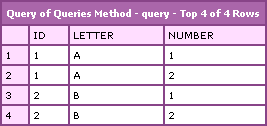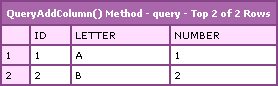# Ask Ben: Adding A Column From Second Query To First Query

By on

I have two queries and I want to add a column from the second query to the first query. What is the best way to do this?

First off, I just want to say that I am not sure why you would want to do something like that, so maybe (likely?) I am misunderstanding what you mean. If you are talking about joining the two queries and selecting all columns from the first and only one column from the second, that can be done using a simple ColdFusion query of queries which supports inner joins and full outer joins. If you are not talking about that, the only other option is that you simply want to add one column, in order, to the other column.

Since I have no idea what you are talking about, let's quickly look at both. But first, let's build two queries with which to experiment:

``````<!--- Create the first empty query. --->
<cfset qOne = QueryNew( "" ) />

<!---
Populate the first query with a column of ids
(think primary key / identifier).
--->
qOne,
"id",
"CF_SQL_INTEGER",
ListToArray( "1,2" )
) />

<!--- Populate the first query with a column of values. --->
qOne,
"letter",
"CF_SQL_VARCHAR",
ListToArray( "A,B" )
) />

<!--- Create the second empty query. --->
<cfset qTwo = QueryNew( "" ) />

<!---
Populate the first query with a column of ids
(think primary key / identifier).
--->
qTwo,
"id",
"CF_SQL_INTEGER",
ListToArray( "2,3" )
) />

<!--- Populate the second query with a column of values. --->
qTwo,
"number",
"CF_SQL_VARCHAR",
ListToArray( "1,2" )
) />
``````

Now we have two queries: one has a column of letters and the other has a column of numbers (both have an identifier column in order to mimic a more real-world situation). If we go the query of queries route, it would look something like this:

``````<!---
Join both queries using an outer join (no "ON" where
clause defined and no column to match on). Grab all
columns from the first query and only ONE column (the
one we are adding) from the second column.
--->
<cfquery name="qThree" dbtype="query">
SELECT
qOne.*,
qTwo.number
FROM
qOne,
qTwo
</cfquery>

<!--- Output the resultant cartesian product. --->
<cfdump
var="#qThree#"
label="Query of Queries Method"
/>
``````

Notice that in our ColdFusion query of queries, we are not specifying any join condition. This acts like a FULL OUTER JOIN and produces the cartesian product of all joined query columns:This adds the entire column of the second query to every row of the first query. Not sure how useful this would be to you unless you included a join condition (in the WHERE clause).

If you actually mean just adding appending a column of one query to the existing columns of another query, well, this is quite easy. If you look above at how we created the original test queries, you might get an idea as to how we are going to do this - just add the column:

``````<!---
Add the number column of query two the first query. When
doing this, it is essential that we reference the column
using array notation (ex. [ "column" ]). If we do NOT do
that, then ColdFusion things we are referring to the first
column value and NOT the column itself.
--->
qOne,
"number",
"CF_SQL_VARCHAR",
qTwo[ "number" ]
) />

<!--- Display the results. --->
<cfdump
var="#qOne#"
/>
``````

Note that we added the column using a simple QueryAddColumn() method. ColdFusion rocks so hard core! Also note that I needed to refer to the second query's column using array notation:

qTwo[ "number" ]

This is required. Remember, ColdFusion is used to getting cell values from a query - it's not used to referencing the entire record set column. If you were to add this:

qTwo.number

... to the first query, ColdFusion would assume you are trying to pass in the value "1" which is the first column value. Doing so would throw the error:

Object of type class java.lang.String cannot be used as an array

That being said, performing the above action results in the following output:This outcome here is quite different than the outcome of the ColdFusion query of queries. Using QueryAddColumn() simply appends the column. It doesn't change the order of either query not does it check any join information; it simply adds the column.

Again, I am not sure what exactly you were going for. The ColdFusion query of queries method seems the more useful of the two, but it didn't sound like what you were trying to do. The QueryAddColumn() is quite literal, but as far as I can see, not very useful (in this type of a scenario). But, if you can find a use for it, I would be excited to see it.

Want to use code from this post? Check out the license.I have had to do this with data from databases on different servers because I wanted to use the grouping functions of cfoutput.

I always did it by defining a fake field in the first query like so:

select Field1, '' AS Field2
from Table1

Then, with the data from the second database I looped over it and set the field manually.
<cfloop query="FirstQuery">
<!---Logic here to find the right row in the second query--->
<cfset FirstQuery.Field2 = ValueYouWantFromSecondQuery>
</cfloop>

It may not be the best solution, but it was intuitive and easy.@Lyle,

Yeah, I do that a lot. I actually do that even on a single query - sometimes it's easier to do crazy calculation after the fact. For instance, if I have a query that has two aggregate columns or sub-query columns and then I need a third column that averages them (divides one over the other), I find that it's easier to do (0 AS average) and then do the division after the query runs. Easy AND faster (since I don't have to re-calc the other columns for the average).I'm not able to do an outer join with 2 condtitions.

I have 2 queries

Have you ever been able to do this?